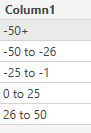cancel
Showing results for
Did you mean:Frequent Visitor

## Period Comparison Growth Category

Dear All,

I really need a help regarding Growth Category based on my growth % (Period Comparison). I have created Period1 and Period 2 measures and I have created a measure for Growth %.  But I want to create Category based on my Growth%. Since Growth% is a measure (Row Context) I coundnt Achieve what am expecting.

I have created two Tables Date 1,

I have created the following measures:

Sales = SUM('demo'[total_sales])
Period 1 = CALCULATE([Sales],KEEPFILTERS(TREATAS(VALUES('Date 1'[invoice_date]),'demo'[invoice_date])))
Period 2 = CALCULATE([Sales],KEEPFILTERS(TREATAS(VALUES('Date 2'[invoice_date]),'demo'[invoice_date])))
Growth % = IF([Period 1]<>0,([Period 2]-[Period 1])/[Period 1],
IF(AND([Period 1]=0,[Period 2]=0), 0,
IF(AND([Period 1]=0,[Period 2]<>0),[Period 2],
IF([Period 1]=[Period 2],0
)
)))

This is what I want to achieve to see how many stores falls into the respective category. The below mentioned Table is the one am expecting.
Growth Category = IF([Growth %] < -0.50, "-50+",
IF(AND([Growth %] < -0.25,[Growth %] >= -0.50), "-50 to -26",
IF(AND([Growth %] < 0,[Growth %] >= -0.25), "-25 to -1",
IF(AND([Growth %] > 0,[Growth %] <= 0.25), "0 to 25",
IF(AND([Growth %] > 0.25,[Growth %] <= 0.50), "26 to 50",
IF([Growth %] > 0.50, "51+",
IF([Growth %] = 0.00, "Zero Growth", "Zero Sales"
)
))))))
 Growth Category -50+ -50 to -26 -25 to -1 0 to 25 26 to 50 50+ No.of.Stores 7 15 51 8 12 5

Thank you.

2 REPLIES 2Community Support

Hi, @Beniya_Samson ;

You could change the [Growth Category ] to column or you need add a new table like this.If the above one can't help you get the desired result, please provide some sample data in your tables (exclude sensitive data) with Text format and your expected result with backend logic and special examples. It is better if you can share a simplified pbix file. You can refer the following link to upload the file to the community. Thank you.

How to upload PBI in Community

Best Regards,
Community Support Team _ Yalan Wu
If this post helps, then please consider Accept it as the solution to help the other members find it more quickly.Super User

@Beniya_Samson , Are you trying something like that

Power BI Abstract Thesis: How to use two Date/Period slicers

https://youtu.be/WSeZr_-MiTg

!! Microsoft Fabric !!
Microsoft Power BI Learning Resources, 2023 !!
Learn Power BI - Full Course with Dec-2022, with Window, Index, Offset, 100+ Topics !!
Did I answer your question? Mark my post as a solution! Appreciate your Kudos !! Proud to be a Super User! !!